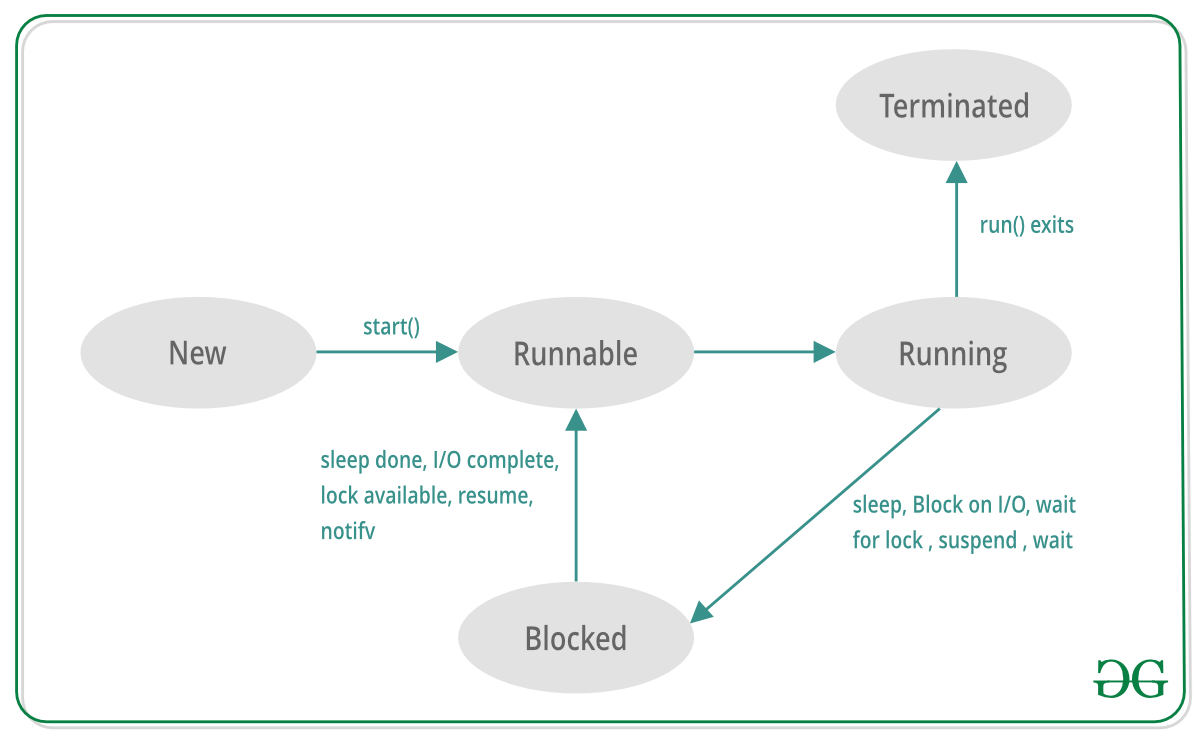Open in App
Not now

• Last Updated : 08 Apr, 2019

A process in which multiple threads executing simultaneously that is called multithreading. It allows you to perform multiple tasks independently.

Threads are lightweight sub-processes which occupy less memory. A multi-threaded program contains two or more threads that can run concurrently and each thread can handle a different task at the same time making optimal use of the available resources specially when your system(computer) has multiple CPUs. Multithreading is used to develop concurrent applications in Scala.

Threads in Scala can be created by using two mechanisms :

2. Extending the Runnable Interface

We create a class that extends the Thread class. This class overrides the run() method available in the Thread class. A thread begins its life inside run() method. We create an object of our new class and call start() method to start the execution of a thread. Start() invokes the run() method on the Thread object.

 `// Scala code for thread creation by extending  ` `// the Thread class  ` `class` `MyThread ``extends` `Thread  ` `{ ` `    ``override` `def` `run() ` `    ``{ ` `        ``// Displaying the thread that is running  ` `        ``println(``"Thread "` `+ Thread.currentThread().getName() +  ` `                                        ``" is running."``) ` `    ``} ` `}  ` ` `  `// Creating object ` `object` `GFG ` `{ ` `    ``// Main method ` `    ``def` `main(args``:` `Array[String]) ` `    ``{ ` `        ``for` `(x ``<``- ``1` `to ``5``)  ` `        ``{ ` `            ``var` `th ``=` `new` `MyThread() ` `                        ``th.setName(x.toString()) ` `                            ``th.start() ` `        ``} ` `    ``} ` `} `

Output :

```Thread 1 is running.
```
• Thread creation by Extending Runnable Interface

We create a new class which extends Runnable interface and override run() method. Then we instantiate a Thread object passing the created class to the constructor. We then call start() method on this object.

 `// Scala code for thread creation by implementing  ` `// the Runnable Interface  ` `class` `MyThread ``extends` `Runnable  ` `{ ` `    ``override` `def` `run() ` `    ``{ ` `        ``// Displaying the thread that is running  ` `        ``println(``"Thread "` `+ Thread.currentThread().getName() + ` `                                            ``" is running."``) ` `    ``} ` `}  ` ` `  `// Creating object ` `object` `MainObject ` `{ ` `    ``// Main method ` `    ``def` `main(args``:` `Array[String]) ` `    ``{ ` `        ``for` `(x ``<``- ``1` `to ``5``)  ` `        ``{ ` `            ``var` `th ``=` `new` `Thread(``new` `MyThread()) ` `                        ``th.setName(x.toString()) ` `                            ``th.start() ` `        ``} ` `    ``} ` `} `

Output :

```Thread 1 is running.
```

Note : threads need not be running in any sequential order. All the threads run concurrently and independent of each other.

In between the period of creation and Termination of a Scala Thread, the thread undergoes various state changes. These constitute the Life Cycle of a Scala Thread. It has the five following states.

• New : This is the first state when the Thread is just created.
• Runnable : This is the state when the Thread has been created but the Thread has not got the chance to start running.
• Running : In this state the Thread is performing its task.
• Blocked (or Waiting): This is the state when the thread is still alive, but is currently unable to run due to waiting for input or resources.
• Terminated : A thread is in dead state when its run() method exits.My Personal Notes arrow_drop_up
Related Articles